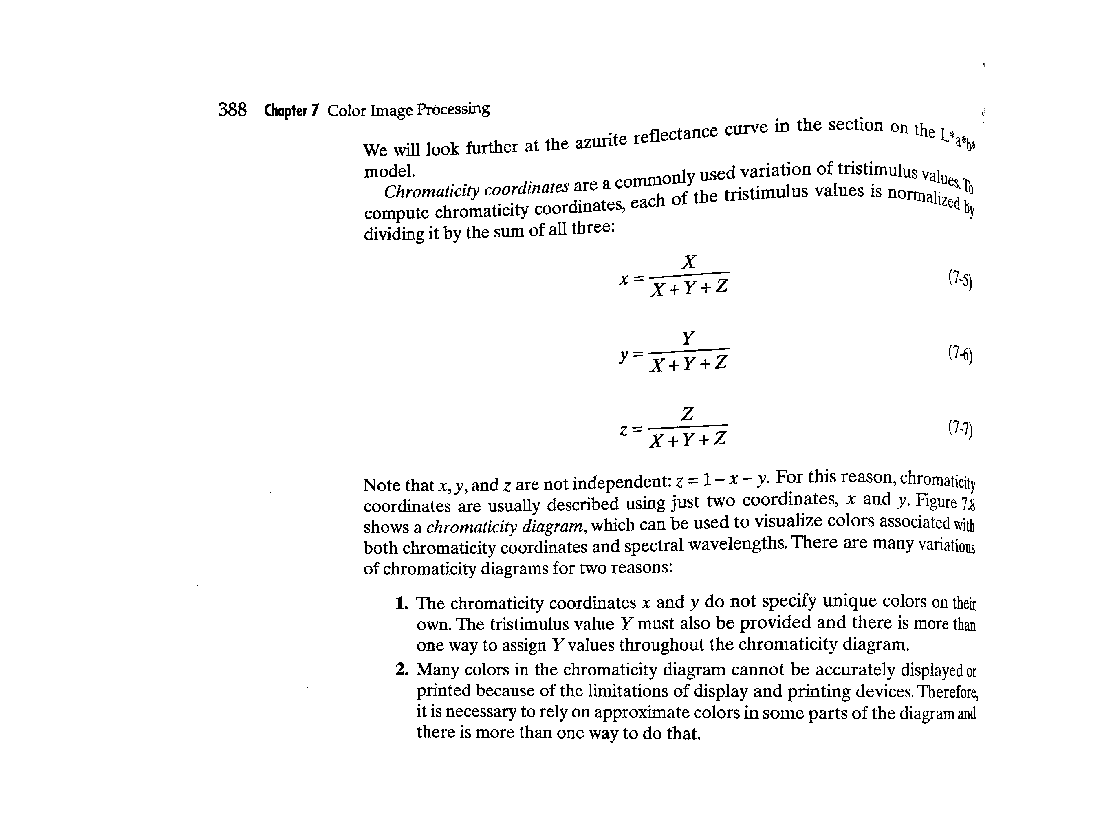# Use imbinarize to Threshold Gray-Scale Images

Today, I want to convince you to use imbinarize instead of im2bw.
Background: I recently saw some data suggesting that many Image Processing Toolbox users are still using im2bw, an old function that dates back to the original toolbox release in 1993. We recommend using the newer function, imbinarize, because it saves a step in the most common scenario, and because it offers additional flexibility if you need it.
I'll explain by updating an old blog post topic called "Tracing George," in which I try to extract the curve shown in the following image:
url = 'https://blogs.mathworks.com/images/steve/36/george.jpg';
imshow(I)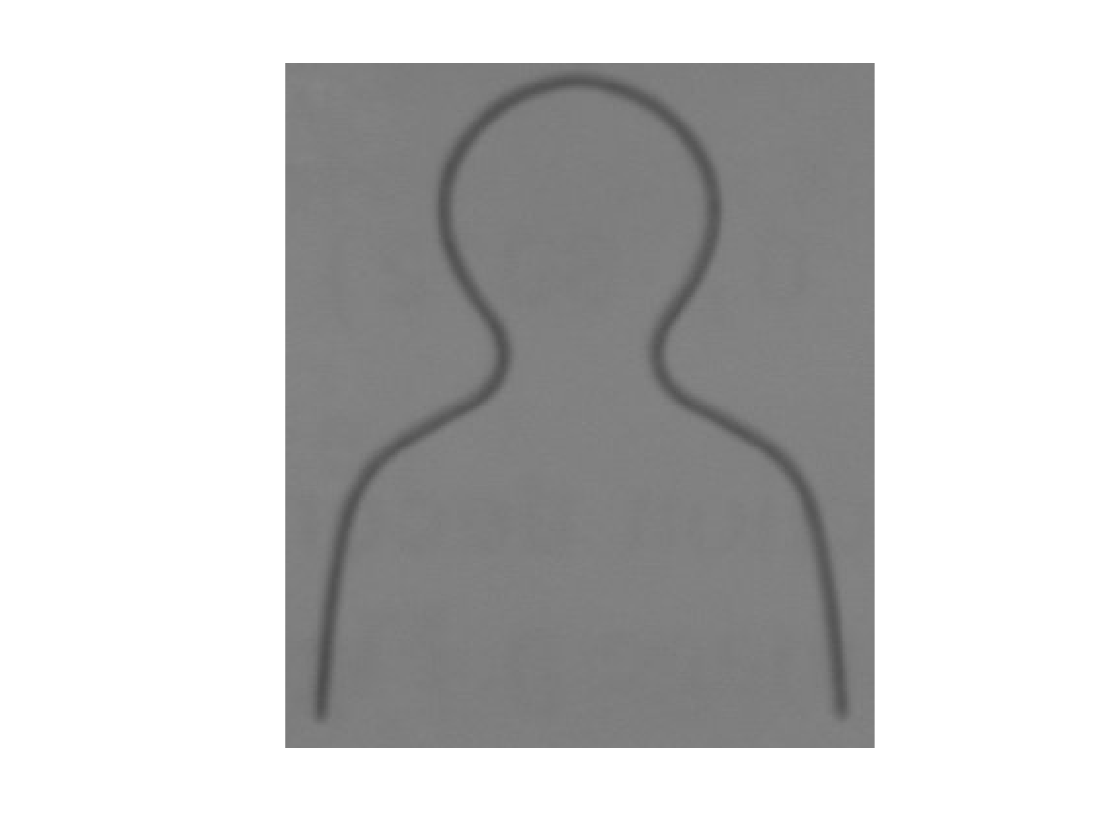I call this "George." I created George originally for some examples and diagrams in the book Digital Image Processing Using MATLAB. I found at some point that I needed to reproduce the outline curve, but I didn't have the original curve data. (Silly me.) So, I experimented with ways to extract the original curve data from the image.
An obvious first step is to threshold the image. In my original blog post, I used two functions together:
threshold = graythresh(I);
bw = im2bw(I,threshold)
The function graythresh is used to compute an "optimal" threshold value (optimal according to certain criteria). The function im2bw can be used without specifying the second argument, but then it just uses a fixed threshold value that usually isn't satisfactory:
bw = im2bw(I);
imshow(bw)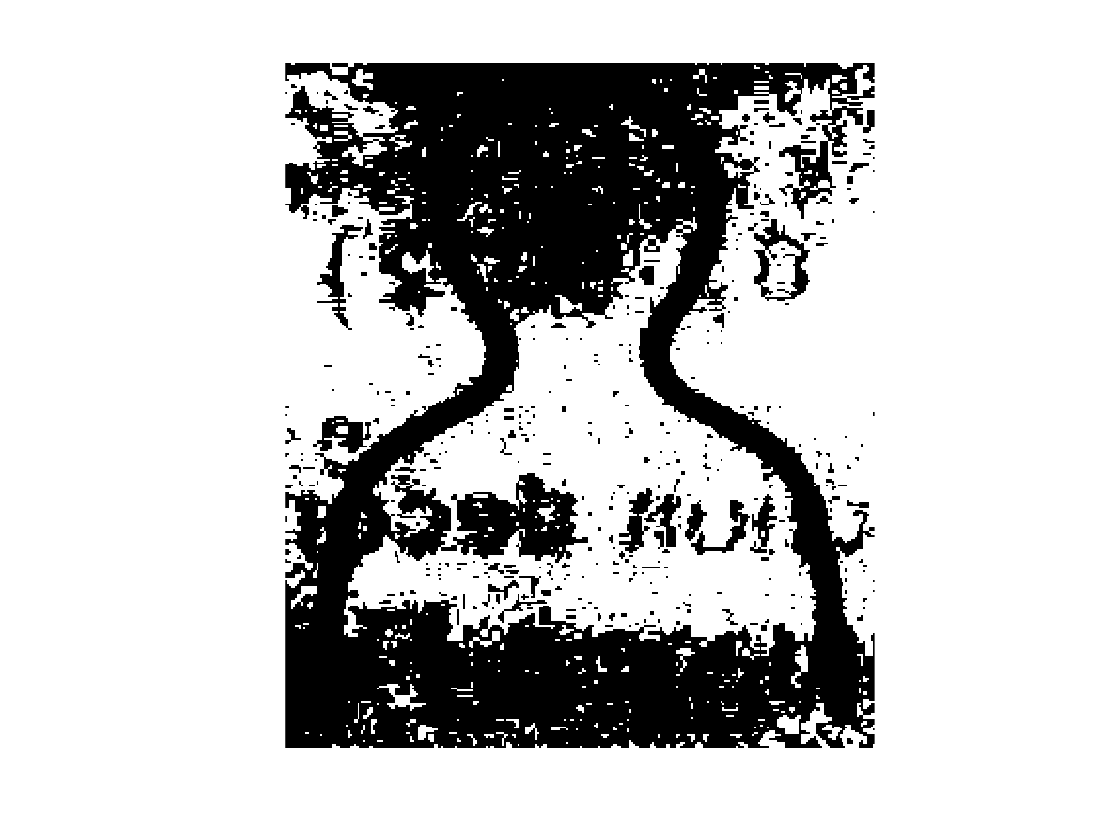The result with graythresh is much better.
t = graythresh(I)
t = 0.4392
bw2 = im2bw(I,t);
imshow(bw2)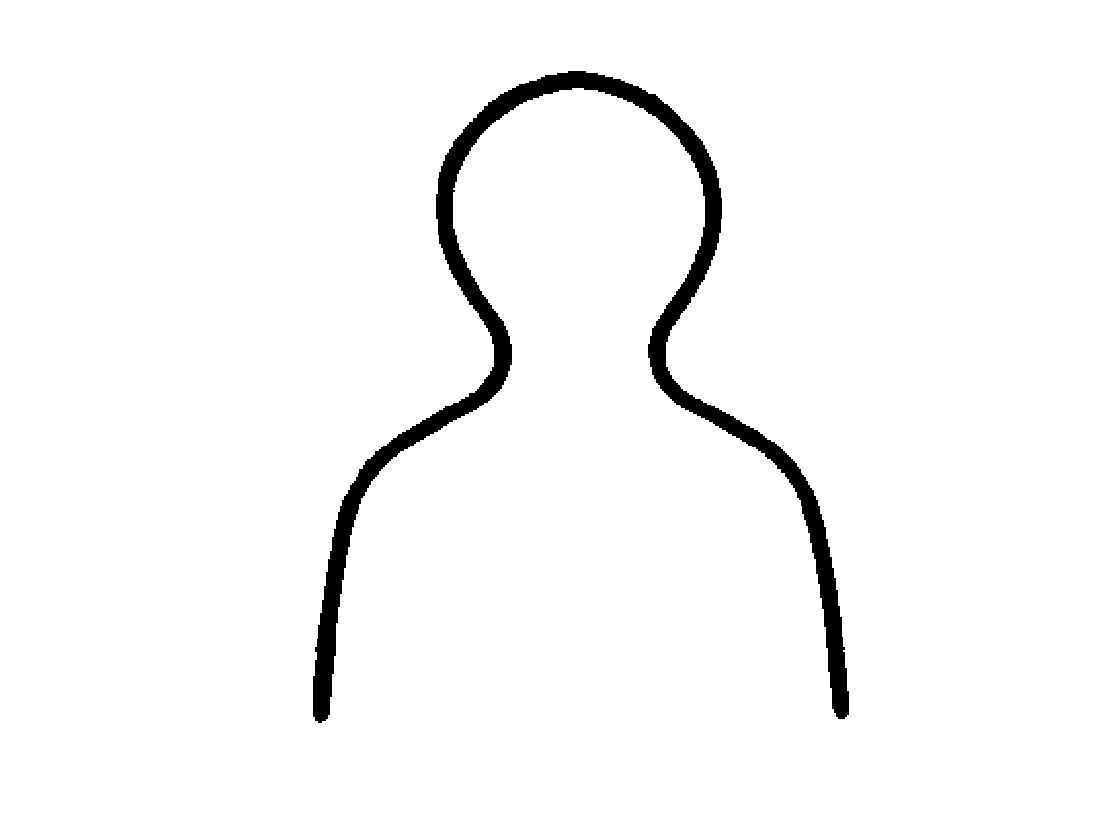Over time, we observed that almost everyone (including us) always used im2bw and graythresh together. That caused to reconsider the functional design. The replacement function, imbinarize, uses graythresh automatically.
bw3 = imbinarize(I);
imshow(bw3)As a side note, here's the rest of the code that I used to extract the curve. See my original "Tracing George" blog post for an explanation.
bw4 = ~bw3;
bw5 = bwmorph(bw4, 'thin', inf);
boundaries = bwboundaries(bw5);
b = boundaries{1};
b = b(1:floor(end/2), :);
x = b(:,2);
y = b(:,1);
plot(x,y)
axis ij
axis equal
title('George P. Burdell')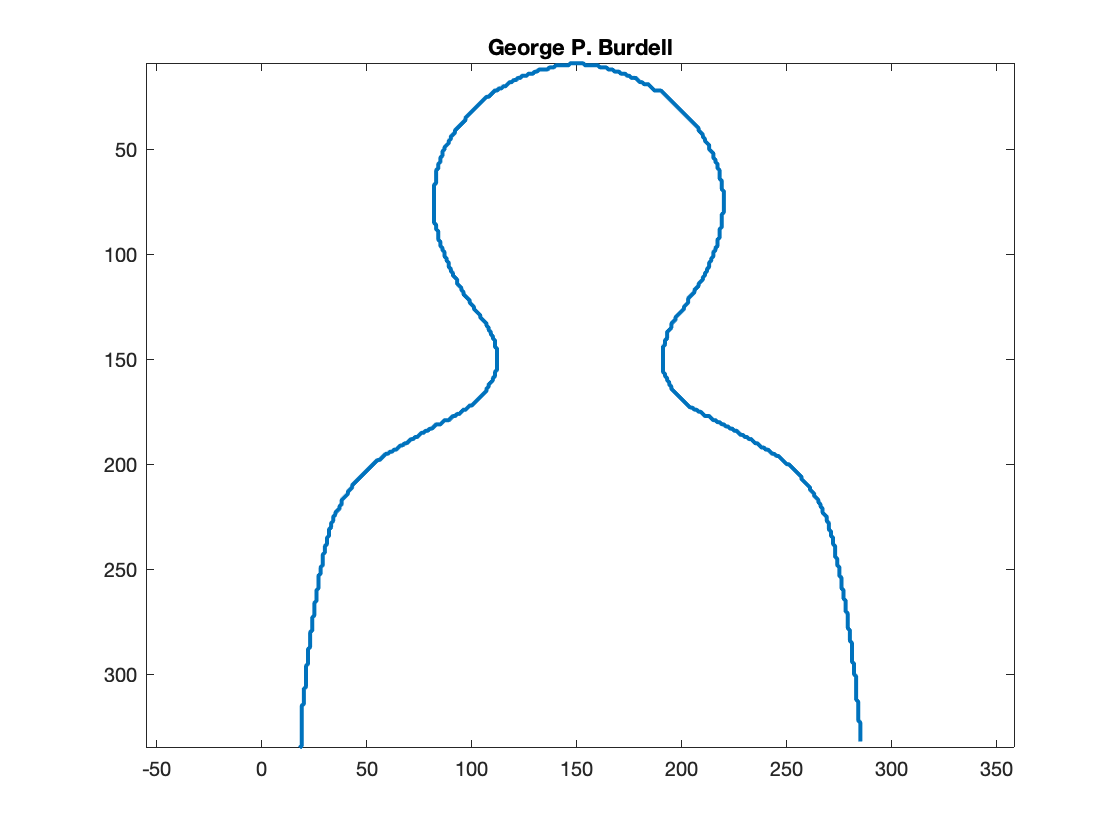For more difficult thresholding challenges, imbinarize also supports a locally adaptive method. To demonstrate, here's a picture of a page from Digital Image Processing Using MATLAB that I took in my office just now, with uneven lighting.
page_url = "https://blogs.mathworks.com/steve/files/dipum3e-page-388-gray.jpg";
imshow(A)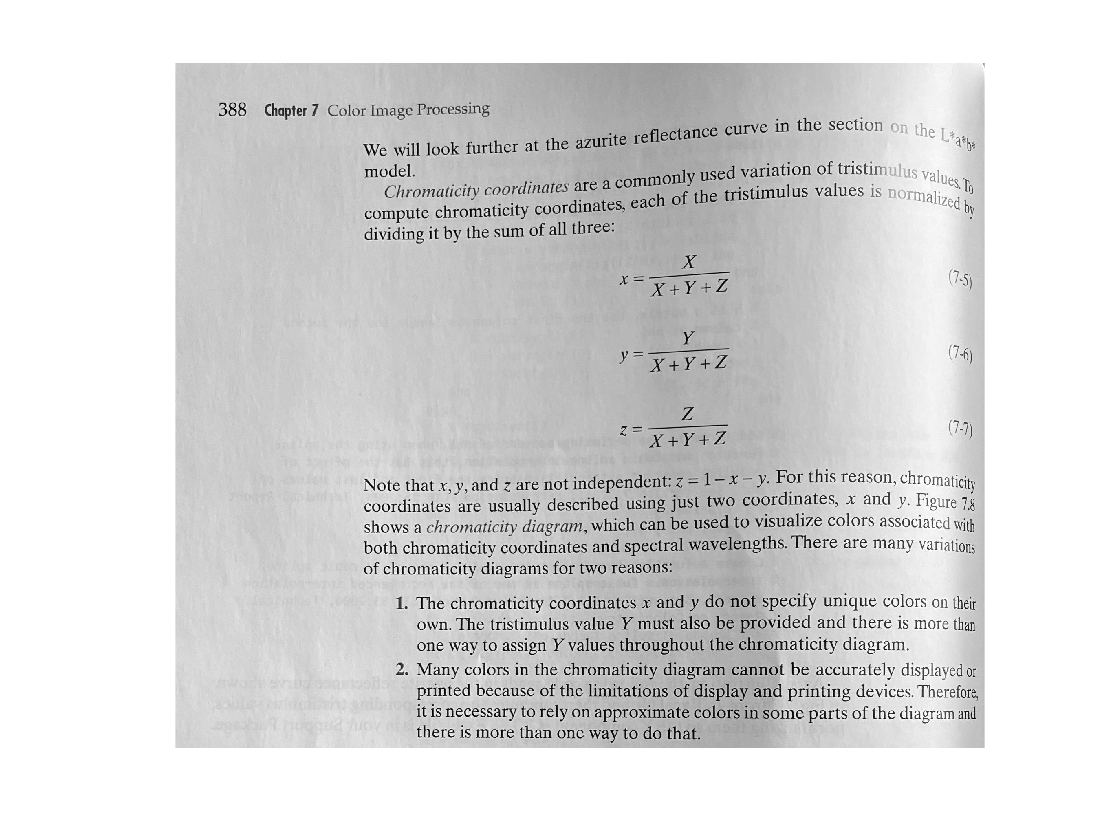Because of the uneven lighting, even the best global threshold doesn't produce a suitable result.
page_bw = imbinarize(A);
imshow(page_bw)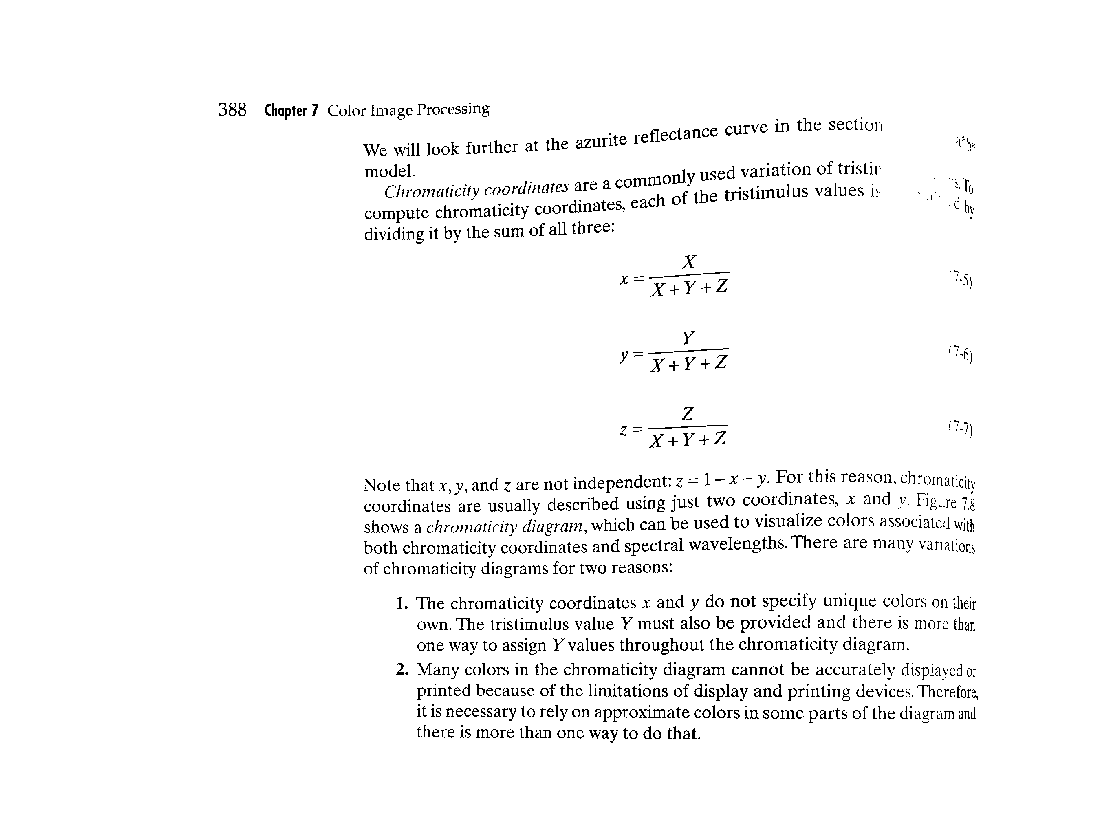You can see that there is an area of missing text at the upper right. A locally adaptive thresholding method would be better. The function imbinarize supports a locally adaptive method, which is specified using the "adaptive" input option. With locally adaptive methods, though, it is very helpful to specify whether the foreground lighter or darker than the background. You can tell imbinarize that information using the "ForegroundPolarity" option.
Here it is in action.